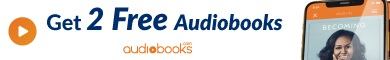# Script doesnt work#### chris4321234

##### Active Member
Hello everyone, I tried to make a Script, with a default SL, TP for Limit Orders and saved it in the right script ordner, but it doesnt work.
Can someone find an error, because I dont see one?

extern int PipSL = 35;
extern int PipTP = 90;
extern double Lots = 0.1;
extern int Order = 1; //BuyLimit
extern int Distance = 15; //Pending Distance to current Price
extern int PendingTime = 6000;
extern int Slippage = 5;
extern int Magic = 666;

void OnStart()
{
//---

double SL;
double TP;
int Pip = 0.0001;
if (Digits == 2 || Digits == 3) {Pip = 0.01;}
double SellEntry = Bid+(Distance*Pip);

if (Order == 1) {
SL = NormalizeDouble(BuyEntry - (PipSL * Pip),Digits);
TP = NormalizeDouble(BuyEntry + (PipTP * Pip),Digits);

if (Order == -1) {
SL = NormalizeDouble(SellEntry + (PipSL * Pip),Digits);
TP = NormalizeDouble(SellEntry - (PipTP * Pip),Digits);
OrderSend(Symbol(),OP_SELLLIMIT,Lots,SellEntry,Slippage,SL,TP,NULL,Magic,PendingTime,Red);}
}

#### jack

Staff member
(I know this is an older post, but..)

Few quick things:

1) Wrap your code with the
Code:
`````` BB tags so we can see it presented with easy to read indentation and such. Looks like this:

if (Order == 1) {
SL = NormalizeDouble(BuyEntry - (PipSL * Pip),Digits);
TP = NormalizeDouble(BuyEntry + (PipTP * Pip),Digits);